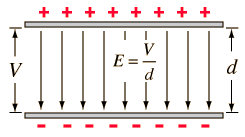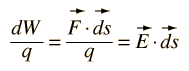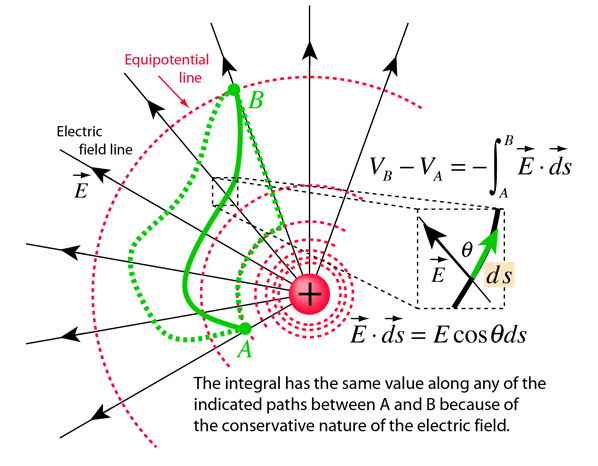# Work and Voltage: Constant Electric Field

 The case of a constant electric field, as between charged parallel plate conductors, is a good example of the relationship between work and voltage.The electric field is by definition the force per unit charge, so that multiplying the field times the plate separation gives the work per unit charge, which is by definition the change in voltage.This association is the reminder of many often-used relationships:More general case
Index

Voltage concepts

Electric field concepts

 HyperPhysics***** Electricity and Magnetism R Nave
Go Back

# Voltage Difference and Electric Field

The change in voltage is defined as the work done per unit charge against the electric field. In the case of constant electric field when the movement is directly against the field, this can be writtenIf the distance moved, d, is not in the direction of the electric field, the work expression involves the scalar product:In the more general case where the electric field and angle can be changing, the expression must be generalized to a line integral:More detail on variable field
Index

Electric field concepts

Voltage concepts

 HyperPhysics***** Electricity and Magnetism R Nave
Go Back

# Voltage from Electric Field

The change in voltage is defined as the work done per unit charge, so it can be in general calculated from the electric field by calculating the work done against the electric field. The work per unit charge done by the electric field along an infinitesmal path length ds is given by the scalar productThen the work done against the field per unit charge in moving from A to B is given by the line integral:Index

Electric field concepts

Voltage concepts

 HyperPhysics***** Electricity and Magnetism R Nave
Go Back